# 识别猫的单隐藏层神经网络（我的第一个模型）

测试数据50张 64*64

sigmod函数图像：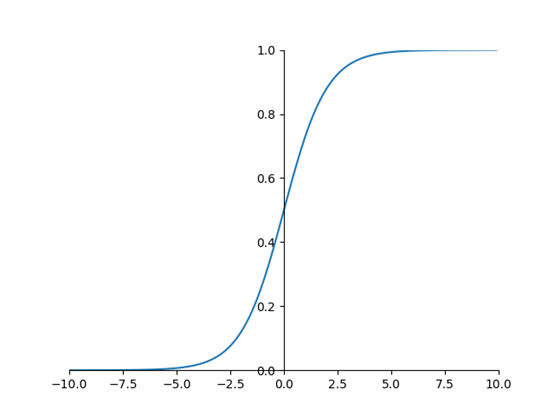ps：logistic回归是算法，但其激活函数取sigmod，是因为，你想得到一个在（0-1）之间的值，所以，这才用到了sigmod函数。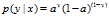log是一个单调递增的函数，可以变成一个凸函数，便于使用梯度下降进行优化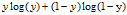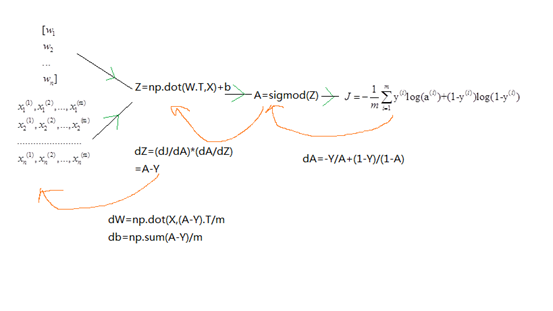1. 载入数据
2. 处理数据（/255），缩小数据，因为1比255更好计算
3. 初始化（w，b），w是一个n维列向量，b=0
4. 建立sigmod函数
5. 利用训练数据集 建立正反向传播函数，如计算图，并求梯度，以便使用梯度下降
6. 建立优化（梯度下降）函数，利用好梯度，选取下降方向，另需选取学习率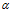，迭代次数。这两个选取与过拟合，欠拟合息息相关
7. 利用迭代num_iterations次后，出来的w，b。组装好sigmod函数，预测，测试集数据
8. 测试/训练集 正确率输出，利于判断
9. 可视化输出，比较出一个好的学习率，和迭代次数。（超参数选择，一个无法学习的东西

1. import numpy as np
2. import matplotlib.pyplot as plt
3. import h5py
4. import sys
5. sys.path.append(r'F:\study\assignment2')
6. from lr_utils import load_dataset
7. '''''
8. 数据读取与标准化
9. 读取的x数据是（20964,64,3）的格式
10. 需要将其平铺成（64*64*3,209
11. '''
12. train_x_orig,train_y_orig,test_x_orig,test_y_orig,classes=load_dataset()
13.
14. train_x_flatten=train_x_orig.reshape(train_x_orig.shape,-1).T
15. test_x_flatten=test_x_orig.reshape(test_x_orig.shape,-1).T
16. #上面一定要记得转置，因为reshape成了（mn）的形式
17. #-1，是不用去考虑到底要转换成多少列了，只要是负数就行
18.
19. #然后是将数据缩小，便于计算，因为1255更好计算，反正也不影响计算机结果
20. #标准化数据 rgb值都小于255
21. train_x=train_x_flatten / 255
22. test_x=test_x_flatten / 255
23.
24. train_y=train_y_orig
25. test_y=test_y_orig
26. #上面就把数据整理好了
27.
28. '''''
29. 下面开始写函数了
30. '''
31.
32. #建立sigmod函数
33. def sigmod(x):
34.     return (1/(1+np.exp(-x)))
35.
36. #初始化wb, psw是一个列向量
37.
38. def initialize(n_x):
39.     w=np.zeros(shape=(n_x,1))
40.     b=0
41.     return(w,b)
42.
43.
44. #建立正反向传播函数
45.
46. def propagate(w,b,X,Y):
47.     """
48. 正向传播
49. """
50.
51.     A=sigmod(np.dot(w.T,X)+b)
52.     m=X.shape
53.     cost=(-1/m)*np.sum(Y * np.log(A)+(1-Y) * np.log(1-A))
54.     '''''  ps:尼玛不知道为什这个三点冒号也要按照缩进格式来
55. 反向传播
56. '''
57.     dw=np.dot(X,(A-Y).T)/m
58.     db=np.sum(A-Y)/m
59.
60.     '''''
61. 组装数据
62. '''
63.     grads={'dw':dw,'db':db}
64.     return (grads,cost)
65.
66. #建立优化函数
67.
68. def optimize(w,b,X,Y,num_iterations,learning_rate,print_cost):
69.     costs=[]
70.     for i in range(num_iterations):
71.         grads,cost=propagate(w,b,X,Y)
72.         dw=grads['dw']
73.         db=grads['db']
74.
75.         w=w-learning_rate*dw
76.         b=b-learning_rate*db
77.
78.         #记录误差
79.         if i % 100==0:
80.             costs.append(cost)
81.         if print_cost and i%100==0:
82.             print('迭代次数：%i.误差值：%f' %(i,cost))
83.     #组装数据
84.     params={'w':w,'b':b}
85.     return (params,costs)
86.
87. #建立预测函数
88.
89. def predict(w,b,X):
90.     value=sigmod(np.dot(w.T,X)+b)
91.     Y_prediction=(value>0.5)+0
92.
93.     '''''
94. 对于上一代码详解
95. value>0.5是个判断语句，>0.5会返回True
96. +0.是让布尔值转化成数字，1
97.
98. '''
99.     return Y_prediction
100.
101.
102. #下面是整合所有函数了
103. def model(X_train,Y_train,X_test,Y_test,num_iterations=2000,learning_rate=0.01,print_cost=False):
104.     #先调用X_trainY_train，来训练处wb
105.     m=X_train.shape
106.     w,b=initialize(X_train.shape)
107.     params,costs=optimize(w,b,X_train,Y_train,num_iterations,learning_rate,print_cost)
108.     w,b=params['w'],params['b']
109.
110.     #计算预测值
111.     Y_prediction_train=predict(w,b,X_train)
112.     Y_prediction_test=predict(w,b,X_test)
113.
114.     #比较
115.     print('TrainRightRate:',format(100-np.mean(np.abs(Y_prediction_train-Y_train))*100),'%')
116.     print('TestRightRate:',format(100-np.mean(np.abs(Y_prediction_test-Y_test))*100),'%')
117.
118.     '''''
119. 上面代码详解
120. absY_p-T_r),此处两相比较，相同，预测对了，则详减为0，预测错了，则为1或者-1
121. abs，全部取正
122. mean，平均值，即有多少个+-1在所有数据中，即在所有数据中错误的个数/总数据
123. 我要的是正确率，所以前面要用100来减
124. '''
125.     #数据组装
126.     d={
127.         'costs':costs,
128.         'Y_prediction_train':Y_prediction_train,
129.         'Y_prediction_test':Y_prediction_test,
130.         'w':w,
131.         'b':b,
132.         'learning_rate':learning_rate,
133.         'num_iterations':num_iterations}
134.     return d
135.
136. #d=model(train_x,train_y,test_x,test_y,num_iterations=2000,learning_rate=0.005,print_cost=True)
137.
138.
139. '''''
140. 上面就算是整个模型搭建完了，下面是可视化
141. 表示迭代次数，学习率，与成本函数的关系
142. '''
143.
144. learning_rate=[0.01,0.001,0.0001]
145. models={}
146. for i in learning_rate:
147.     print ("learning rate is: " + str(i))
148.     models[str(i)]=model(train_x,train_y,test_x,test_y,num_iterations=500,learning_rate=i)
149.     print ('\n' + "-------------------------------------------------------" + '\n')
150. for i in learning_rate:
151.     plt.plot(np.squeeze(models[str(i)]['costs']),label=str(models[str(i)]["learning_rate"]))
152. plt.ylabel('cost')
153. plt.xlabel('iterations')
154.
155. legend = plt.legend(loc='upper center', shadow=True)
156. frame = legend.get_frame()
157. frame.set_facecolor('0.90')
158. plt.show()

1. 从另外一个文件中导入py文件中的某个函数

import sys

sys.path.append(r'path')

from lr_utils import load_datasert

2. 记住X要组装成（n,m）矩阵，w是一个（n,1）的列向量

有利于记住使用np.dot()时，哪个放前面，哪个放后面，也 有利于广播时是否需要转置的判断

3. 数据处理

本例子中是将每个元/255，让[0,255],的数据计算，变成了[0,1]的数据计算，加快计算

还有很多其他的数据处理方式，还没学到，多做多学

4. np.reshape(a,-1)中-1的妙用，只要把一个数组的行写出来了，列向量可以使用-1，让计算机自动计算分配，只要是负值就行
5. w-=learning_rate*dw 不知道为什么不能使用，需要常规操作，w=w-learning_rate*dw
6. A and B，两个判断，只要有一个是False，那整个语句就是错的，本例子，使用and来控制是否输出迭代次数与误差
7. 布尔值转数字 让布尔值'+0'就行了，numpy对于判断语句，也是并行运行的
8. 对于同类型的数据，多用字典dict组装，如梯度，初始值
9. np.squeeze()函数，将(5,1,1)转换成(5,)降维处理，有利于运行，也是可视化操作时，作为输入时，不可缺少的操作

>>> a=np.random.randn(10000,1,1,1,1)

>>> sys.getsizeof(a)

80160

>>> b=np.random.randn(10000)

>>> sys.getsizeof(b)

80096

10. 可视化操作中，参数解释：loc：选择把线型说明放在哪个地方，shadow，是否加营养

frame.set_facecolor（'0.9'），给说明的地方加了框之后，再选择填充框的透明度

11. 使用'''作解释时，也需要遵循Python的缩进格式，否则会报错
12. 空一行 print('\n')
posted @ 2018-07-13 21:41 天字三号房 阅读(...) 评论(...) 编辑 收藏